﻿ The Difference in the Improvement of Problem Solving Ability and Students Motivation Who Taught by Problem Based Learning and Discovery LearningPublications are Open
Access in this journal
Article Versions
Export Article
• Normal Style
• MLA Style
• APA Style
• Chicago Style
Research Article
Open Access Peer-reviewed

### The Difference in the Improvement of Problem Solving Ability and Students Motivation Who Taught by Problem Based Learning and Discovery Learning

Verawati , E. Elvis Napitupulu, Waminton Rajagukguk
American Journal of Educational Research. 2018, 6(12), 1609-1617. DOI: 10.12691/education-6-12-4
Received October 18, 2018; Revised November 25, 2018; Accepted December 07, 2018

### Abstract

This study aims to determine: (1) differences in the improvement of mathematical problem solving abilities between students given learning Problem Based Learning (PBL) and guided discovery learning, (2) differences in the increase in student motivation given learning Problem Based Learning (PBL) and guided discovery learning, (3) the process of answering students given learning Problem Based Learning (PBL) and guided discovery learning. This research is a quasi-experimental study. The population of this study was all students of class VII of SMP Negeri 1 Pujud and the sample of this study was students of class VII 2 and VII4, each of which is 30 students. The instruments used consisted of: (1) Tests of mathematical problem solving abilities and (2) Questionnaire of student learning motivation. Data analysis was carried out by analysis of covariance (ANCOVA). The results showed that (1) There were differences in the improvement of mathematical problem solving abilities between students given learning Problem Based Learning (PBL) and students who were given guided discovery learning, (2) There were differences in learning motivation between students who were given learning Problem Based Learning (PBL) with students who were given Guided Discovery learning, and (3) the answer resolution process of students with mathematical problem solving abilities given learning Problem Based Learning (PBL) was better because almost all students could solve the problem by fulfilling the scoring rubric of mathematical problem solving abilities compared to Guided Discovery learning.

### 1. Introduction

Education is a conscious effort to develop the potential of students through learning activities. According to Law No. 20 of 2003 1 concerning the National Education System, Education is a conscious and planned effort to create a learning atmosphere and learning process so that students actively develop their potential. Rajagukguk (2011) 2 said that true education is education that prepares students to become quality human resources. The educational process is carried out in such a way that people can understand and appreciate the meaning of education so that it can be useful for themselves in living life.

Recognizing its important role, mathematics education needs to anticipate the increasingly complex and complex challenges of the future. One of the skills related to the characteristics of high-level thinking and low-level thinking is problem solving ability. The importance of problem solving skills by students in mathematics. Syaiful (2012) 3 as follows: (1) problem solving ability is the general goal of teaching mathematics, even as the heart of mathematics; (2) problem solving includes methods, procedures, and strategies which are core and main processes in the mathematics curriculum; and (3) problem solving is a basic ability in learning mathematics. As an implication of the above opinion, the problem solving ability should be owned by all children who study mathematics starting from the elementary school level to the university level.

Reality in the field, students do not have problem solving skills, students often do not understand the true meaning of the problems given by the teacher. The most experienced difficulty or error is in the strategy of carrying out calculations, checking the process and results of calculations. Astriani, Surya and Syahputra (2017). 4 To express more clearly about mathematical problem-solving abilities, a test was given on rectangular material to class VII students of SMP Negeri 1 Pujud, based on the observation that students 'mathematical problem solving abilities were low, students did not understand the problem seen from students' answers, which directly makes the element known and from the answers the students do not understand the problem, they ignore the information provided so that the problem solving plan does not lead to correct answers. From the results of the answer questions in 30 students only 12 people answered correctly and 18 answered incorrectly. For that problem solving skills in mathematics need to be trained and accustomed to students. This requires students not only as a way to solve mathematical problems but also to solve problems they find in everyday life.

In addition to problem solving skills, the students' learning motivation is also very related. Because motivation has a very important role for students in learning. Dimyanti and Mudjiono (2013: 42) 5 "motivation is the energy that mobilizes one's activities". The low motivation makes students lazy to learn even indifferent in learning mathematics. In the learning process, motivation is one of the most important aspects to consider. Often happens in schools, students who are underachieved are not due to their lack of ability, but due to non-existent motivation, making students exert all their learning abilities.

From the results of preliminary observations of State Junior High School 1 Existing class VII2, amounting to 30 students consisting of 18 male students and 12 female students, obtained data on student motivation which was still low. The low student motivation was observed from indicators: 1) students who were enthusiastic in participating in mathematics learning in the class were only 21.43%, 2) students who did assignments or homework were only 28.57%. It can be concluded that the learning motivation possessed by students is still relatively low.

By looking at the results of the above statement, of course it takes the active role of parents, teachers and the community to improve mathematical problem solving skills and student motivation. Seeing the results of students 'answers to the test questions of students' mathematical problem solving abilities that are still low, as well as learning motivation which is still low from the explanation above, researchers assume from the observation that the mathematics learning process so far in school does not focus on mathematical abilities.

Therefore, one way that can be used by teachers is to establish learning model learning that we can use in an effort to develop both of these abilities, one of the learning models that are thought to be in line with the mathematical characteristics and expectations of the current curriculum is the model learning Problem-Based learning (PBL) with guided discovery.

In accordance with the results of the study Eviyanti, et al (2017) 6 show that "Based on the results of research and discussion, it can be concluded that there are mathematical problem solving abilities that students receive from the application of the model of problem -based learning and conventional learning in the opportunities who received students material in class VII of Banda Aceh 1 State Middle School. The problem solving ability of students through problem-based learning is is better than students who received conventional learning. The same thing was said in the Nupita (2013) study 7 "The application of guided discovery learning models to improve learning outcomes and problem solving skills" stated that the results of the study showed that using a guided discovery model could have a positive effect on learning outcomes and problem solving skills students.

Based on the explanation above, the model Problem Based Learning (PBL)and guided discovery learning are sought to improve problem solving skills and motivation to learn mathematics, because students start working on the problems given, linking the problems to be investigated by reviewing the problem from many subjects, carry out authentic investigations to find real solutions to real problems, make products in the form of reports, demonstrate physical models to other friends, work together with each other to develop social skills and thinking skills. Therefore, the authors feel the need to conduct research to see: "Differences in Increasing the Mathematical Problem Solving Ability and Student Motivation That Are Given aModel Problem-Based Learning (PBL) and Guided Discovery".

### 2. Methods

This study is a quasi-experimental research (quasiexperiment). In this quasi-experimental study, it is not possible to control all external variables that influence the course of the study. And in this study, grouping of experimental class 1 and experiment 2 was carried out based on previously formed groups or existing groups. The treatment in this study is mathematics learning withmodels Problem-Based Learning (PBL) and guided discovery learning, while the observed variables are mathematical problem solving abilities and student motivation. In this design there are two randomly selected groups which are used as experimental class-1 and experimental class-2, as in the following table:

### 3. Results and Discussion

The main purpose of this study was to look at the differences in mathematical problem solving abilities and learning motivation of students of SMP Negeri 1 Pujud which were taught with models Problem Based Learning (PBL)and guided discovery learning. In addition, this study also aims to determine the process of student answers to the model Problem Based Learning (PBL)and guided discovery learning.

3.1. Test Results of Capability Tests Problems Pretest Mathematical and Questionnaires of Student Motivation
3.1.1. Test Results and Post-Test of Capability Ability Mathematical Problems

The results of tests of mathematical problem solving abilities provide information about students' mathematical problem solving abilities before and after the learning process, both in experimental class 1 and in the experimental class -2. The research data in the form of tests were obtained from the results of the pretest and posttest of students' mathematical problem solving abilities and from the N-Gain. N-Gain is calculated with the help of Microsoft Office Exel 2007 by dividing the difference between posttest scores and pretest scores with the difference between ideal scores and pretest scores. Summary results of the descriptive analysis of the data mathematical problem solving ability of students both study groups are presented in Table 2 below:

Based on Table 2 above it was obtained that the increase in learning with the model Problem Based Learning (PBL) before the value learning process the average is 44.64 and after learning becomes 84.76 with normalized N-Gain of 0.81 and the percentage increase of 40.64%. Whereas for guided discovery learning the average value before learning is 42.44 and after learning becomes 77.14 with normalized N-Gain of 0.71 and an increase in percentage of 34.7%. To improve the problem solving abilities of students who get learning with the model Problem Based Learning (PBL) included in the category of high N-Gain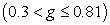which is 0.81, while for the improvement of mathematical problem solving abilities students who get guided discovery learning also included in the category of High N-Gain which is 0.71.

The description of the average increase in problem solving skills based on learning presented in Table 2 can be seen in Figure 1 below.

• Figure 1. Differences in Enhancement Problem Solving Capabilities

Based on Figure 1 obtained information that the average score of the increase in the pretest of mathematical problem solving abilities of students using Problem Based Learning (PBL) and guided discovery learning experienced an increase in the average score of posttest mathematical problem solving abilities of students using Problem Based Learning (PBL) and learning guided discovery. While learning Problem Based Learning (PBL) and guided discovery learning also experienced an increase in N-Gain in students' mathematical problem solving abilities.

3.1.2. Description of Questionnaire Test Results Motivation for Experimental Learning 1 and Experimental Class-2

The results of the student learning motivation questionnaire test provide information about student learning motivation questionnaires before and after the learning process, both in experimental class-1 and in experimental class-2. The research data in the form of tests were obtained from the results of the pretest and posttest student learning motivation questionnaire as well as from the N-Gain. N-Gain is calculated with the help of Microsoft Office Exel 2007 with questionnaire data on students' learning motivation both learning groups are presented in the following Table 3.

Based on Table 3 obtained that the increase learning with themodel Problem Based Learning (PBL)before the learning process the average value is 44.64 and after learning becomes 88.2 with normalized N-Gain of 0.79 and the percentage increase of 43.56%. Whereas for guided discovery learning obtained the average value before learning is 42.44 and after learning becomes 77.13 with normalized N-Gain of 0.64 and the percentage increase of 34.69%. To increase the learning motivation questionnaire students who get learning with themodel Problem Based Learning (PBL)fall into the category of high N-Gain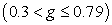which is 0.79, while for the increase in the motivation to learn mathematical learning students who get guided discovery learning also included in the medium N-Gain category which is 0.64.

The description of the average increase in learning motivation questionnaire based on learning presented in Table 3 can be seen in Figure 2 below.

• Figure 2. Difference Bar Diagram Increased Student Learning Motivation Questionnaire

Based on Figure 2 above, information is obtained that there is an increase in the average N-Gain in student learning motivation before the learning process and after learning Problem Based Learning (PBL) and discovery learning guided.

3.2. Inferential Statistical Analysis (ANACOVA) Ability to Solve Problems and Questionnaires Student Learning Motivation
3.2.1. Test Data Normality Ability to Solve Problems and Questionnaires Student Learning Motivation

The formulation of hypotheses to test data normality is:

H0: samples come from populations that are normally distributed

H1: samples come from populations who are not normally distributed.

Testing criteria used is if the value of significance (sig.) Is greater than α = 0.05 thenH0 is accepted. Data normality test used the Kolmogorov-Smirnov test.

Based on Table 4 above shows that the normality test with the Kolmogrov-Smirnov and Shapiro-Wilk test shows that the experimental class pretest score-1 significance value is 0.070>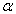: 0.05 and for the experimental class-2 0.132>: 0.05. So thataccepted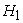rejected. Thus the data pretest the mathematical problem solving abilities of students are normally distributed.

Based on Table 5 above shows that the normality test with the Kolmogrov-Smirnov and Shapiro-Wilk test is known that the experimental class pretest score-1 significance value is 0.2>: 0.05 and for the experimental class-2 0.089>: 0.05. So that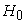acceptedrejected. Thus the data pretest the mathematical problem solving abilities of students are normally distributed.

From the results of the Kolmogorov-Smirnov test, it is known that the significance value of the experimental class 1 is 0.72 and the experimental class 2 is 0.58 where 0.72> α: 0.05 and 0.58> α: 0, 05 so that the data on student motivation in the experimental class 1 and experiment 2 are normally distributed. Thus the data on student motivation as a whole can be concluded to be normally distributed.

3.2.2. Homogeneity Test Data Problem Solving Ability and Questionnaire Student Learning Motivation

Testing homogeneity of the results of pretest and posttest mathematical problem solving ability and student motivation in the experimental class 1 and experimental class 2 aims to determine whether the sample data obtained comes from populations that are homogeneous or not. Results of homogeneity test test mathematical problem solving ability of students in the two classes are presented in Table 7 below:

Based on Table 7 above shows that the significant results of the pretest of mathematical problem solving abilities in the two experimental classes amounted to 0.831 where 0.831>: 0.05. So thatacceptedrejected. Thus the variance of the results of pretest mathematical problem solving abilities of students in the experimental class 1 and experiment 2 came from populations that had the same variance.

Similarly, in Table 8 with the results postes mathematical problem solving ability of students in both the experimental class of 0.178, so it has the Sig> 0.05, thenacceptedrejected. Thus the variance of the posttest results of mathematical problem solving abilities of students in the experimental class 1 and experiment 2 came from populations that had the same variance.

The homogeneity test results of student learning motivation in the two classes are presented in the following:

From the results of the Levene homogeneity test using the program SPSS 20 above, it is known that with the Levene test the significance value is 0.662> α: 0.05 so the null hypothesis is accepted which means that all populations have the same / homogeneous variance. So student learning motivation has the same variance.

3.2.3. Hypothesis Test

To test the difference between two learning class regression models Problem Based Learning (PBL)and guided discovery learning class used variance analysis using F. statistics Statistically the hypothesis can be formulated: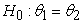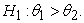Results of calculation of similarity test and coefficient of mathematical problem solving ability learning class Problem Based Learning (PBL) and the guided discovery learning class is described in Table 10 and Table 11 below:

From ANACOVA or test F test, for mathematical problem solving skills studentslearning classes Problem Based Learning (PBL)and guided discovery learning classes were obtained for mathematical problem solving abilities F*= 6.173 based on table F, for α = 5% obtained by F(1-α; 1, n-2) = F(0.95; 1.58) = 4.01 . Because F*>F(0.95; 1.58) and the probability (0,000) is smaller than 0.05 then H0 is rejected and accepted H1. This means that the two linear regression models are not significantly or significantly different.

For the results of covariance analysis using F statistics with predefined formulas and criteria can be seen in Table 12 below:

Based on the results of the covariance analysis for the model obtained Fcount 4.089> Ftable = 4.01 and with sig = 0.048. Because the level of sig less than 0.05, so that H0 is rejected H1 received, it can be concluded there are differences in mathematical problem solving among students by learning Problem Based Learning (PBL) with guided discovery learning. For mathematical problem solving obtained a significant value of 0.05 pretest is smaller, it can be concluded that at the 95% confidence level, the results of mathematical problem solving are influenced by the pretest ability of students before being given learning Problem Based Learning (PBL) with guided discovery learning. Therefore, errors can be corrected by the pretest value as a covariate / variety.

The regression model that has been obtained for mathematical problem solving abilities for Problem Based Learning (PBL) is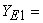65.484 + 0.272XE1 and the equation for guided discovery learning is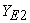=44.064+ 0.68XE2. Subsequent calculations because both regressions for both homogeneous groups and linear regression line constants for mathematical problem solving abilities of learning groups Problem-Based Learning (PBL)of 65.484 are greater than the linear regression equation guided discovery learning group of 44.064 then geometrically regression lines for learning classes Problem-Based Learning (PBL) are above the guided discovery learning regression line.

This indicates that there are differences in the activities of the two regression lines which are influenced by the regression constants. The height of the regression line illustrates student learning outcomes, namely when X = 0 the regression equation for mathematical problem solving ability Problem Based Learning (PBL)65,484 and the guided discovery class regression equation= 44,064. It means that it can be concluded that there are significant differences in mathematical problem solving abilities between students given higher Problem-Based Learning (PBL) learning compared to mathematical problem solving abilities of students given guided discovery learning on quadrilateral material.

Whereas to test the difference between two learning class regression models Problem Based Learning (PBL) and the guided discovery learning class on the learning motivation questionnaire used analysis of variance using F statistics with the formula:The calculation results of the similarity test and the questionnaire coefficient of student learning motivation learning class Problem Based Learning (PBL)and the guided discovery learning class is described in Table 13 and Table 14 below:

From ANACOVA or F test, for student learning motivation learning class Problem Based Learning (PBL) and guided discovery learning classes obtained learning motivation F*= 2.558 based on table F, for α = 5% obtained by F(1-α; 1, n-2) = F(0.95; 1.58) = 4.01. Because F*<F(0.95; 1.58) and probability (0.013) is much smaller than 0.05, H0 is rejected and accepted H1. This means that the two linear regression models are not significantly or significantly different.

For the results of covariance analysis using F statistics with the formulas and criteria that have been determined with the help of program SPSS 20 can be seen in Table 15 below.

Based on the results (Table 15) of the covariance analysis for the model obtained Fcount 7.303> Ftable = 4.01 and with sig = 0.009. Because the sig level is smaller than 0.05, so H0 is rejected H1 is accepted. So it can be concluded that there are differences in learning motivation between students given learning Problem Based Learning (PBL) with guided discovery learning. For learning motivation obtained a significant value of 0.05 pretest is smaller, it can be concluded that at a confidence level of 95%, the results of learning motivation are influenced by students' pretest ability before being given learning Problem Based Learning (PBL)and learningguided discovery. Therefore, errors can be corrected by the pretest value as a covariate / variety.

The regression model that has been obtained for the previous learning motivation questionnaire, namely for learning Problem Based Learning (PBL) is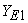= 75,836 + 0,596 XE1 and the equation for the guided discovery learning class is=57,670 + 1,050XE2. Subsequent calculations because both regressions for both homogeneous groups and linear regression line constants for student learning motivation learning group Problem Based Learning (PBL) amounted to 75,836 greater than the linear regression line guided discovery learning group of 57,670 then geometrically regression lines for learning classes Problem Based Learning (PBL) is above the guided discovery learning regression line.

This indicates that there are differences in learning motivation possessed by students both from within and from outside (environment), according to the results obtained means that it can be concluded that learning motivation between students given learning Problem Based Learning (PBL) is higher when compared with students who are given guided discovery learning. With learning Problem Based Learning (PBL) behavior changes occur, due to the impulse that arises in students which results in increased student motivation.

3.2.4. Analysis of Student's Answer Process

Based on the student's answer sheet concerning the test of mathematical problem-solving ability, a general description is obtained that students' answers to the model Problem Based Learning (PBL)and guided discovery learning varies. More fully, the following are the results of tests of mathematical problem solving abilities categorized into indicators of items, namely: students know the elements that are known and asked, students can arrange mathematical problems or develop mathematical models, and students can apply strategies to solve problems.

This problem measures the ability of students with aspects of students to know the elements that are known and asked, students can arrange mathematical problems or develop mathematical models, students can apply strategies to solve problems.

The following is the student's answer process for each class:

The process of solving students' experimental answers 1.

• Figure 3. Students 'Answer Processes in Experimental Classes-1

The picture above is an example of students' answers taught with the model Problem Based Learning (PBL).

The process of solving students' experimental answers 2

• Figure 4. Proses Jawaban Siswa di Kelas Eksperimen-2

The picture above is an example of students' answers taught with guided discovery learning, students do the stages of the problem solving process well because there are some students do not make it known and asked, there are those who do not make a solution, and some do not make a solution.

3.2.5. Results of Analysis of the Process of Completion Student Answers

The process of completing the answers in the experimental class 1

• In the questions number 1 point a, for points a students clearly write information well.

• For point b, and c students have understood the stages of completion according to the mathematical model and can explain the completion procedure well.

The process of completing the answers in the experimental class 2

• For questions number 1 point a, for points a students clearly write information well.

• For point b, and c students have understood the stages of completion according to the mathematical model and can explain the procedure for completion

3.3. Discussion

As for the description of several factors related to this study are as follows:

3.3.1. Problem Solving Mathematical

Ability Students' mathematical problem solving abilities can be measured through student's ability to solve a problem using indicators of mathematical problem solving, namely: Students can identify the elements that are known, and who are asked, students can formulate mathematical problems or develop mathematical models, and students can apply strategies to solve problems.

Based on the results of the study, the average posttest mathematical problem solving ability given learning Problem Based Learning (PBL) amounting to 84.76 higher than students who were given guided discovery learning at 77.14. The results of this study indicate that the average posttest of mathematical problem solving abilities given by Problem Based Learning (PBL) is higher than students who are given guided discovery learning. This is because the learning of Problem Based Learning (PBL), the teacher generates opportunities for students to solve problems in the form of problems, activate students in learning, motivate students to express their ideas and opinions by discussing with their respective groups and then discuss with other groups.

Judging from the ability of the ability indicator, then for each indicator average score mathematical problem solving ability of the experimental class students-1 on the indicator 1 students can identify the elements that are known and asked, all students get a score of 4 as many as 30 students or 100%, while in the experimental class 2, those who got a score of 4 were 28 students or 93.33, students who got a score of 3 were 2 students or 6.67%. For Indicators 2 students can arrange mathematical problems or compile mathematical models in the experimental class - 1 student who scores 4 as many as 17 students or 56.67%, students who get a score of 3 as many as 13 students or 43.33%, while in the experimental class 2 students who scored 4 as many as 13 students or 43.33%, students who scored 3 as many as 17 students or 56.67%. and for indicators c students can apply strategies to solve problems in experimental class-1 get a score of 6 as many as 4 students or 13.33%, score 5 as many as 15 students or 50%, score 4 as many as 11 students or 36.67%, while in class experiment-2 students who scored 5 as many as 17 students or 56.67%, scores 4 as many as 13 students or 43.33%. From each indicator of mathematical problem solving above it can be concluded that the experimental class-1 is higher than the experimental class-2. This is because the best indicators achieved by students after the application of learning Problem Based Learning (PBL) can solve a mathematical problem.

Based on the results of the covariant analysis data shows that there are differences in the increase in mathematical problem solving skills significantly between students taught with learning models with Problem Based Learning (PBL)guided discovery learning, Mathematical problem solving skills that get learning Problem Based Learning (PBL) are higher than those who are given learning guided discovery. This difference can be a reference for decision making that mathematical problem solving abilities in learning classes Problem Based Learning (PBL) higher than guided discovery learning. These findings are also reinforced by the results of the research of Amalia, Surya and Syahputra (2017) 8 showing the average value of students taught using PBL is higher than students taught by using conventional learning modes. This means that learning using PBL is effective in improving mathematical problem solving skills for students. The same thing was said by researchers Syahputra & Surya (2017) 9 "The development of learning based models on problem solving to construct high-order thinking skills in mathematics of 11th grade in SMA / MA". Stating that a concrete learning model can be implemented to improve students' high-level thinking skills in solving mathematical problems. The results of the trial showed a significant increase in students' mathematical problem solving abilities.

From the explanation above shows that learning Problem Based Learning (PBL) has a greater influence in developing skills problem solving. This is due to several things, one of which is the characteristics of learning PBL can encourage students to conduct their own evaluations of both the results and the learning process, and can provide opportunities for students to apply the knowledge they have to other groups. In addition, at the beginning of learning the first meeting in the experimental class 2 students need a long time to follow the mathematical stages found in LAS students, this is because students are not accustomed to solving problems with mathematical stages found in LAS, but students continue to experience progress that is getting better in the process of thinking individually and in groups. Through the stages in LAS understanding mathematical problems by just observing the problem solving problems initially feels difficult for students because they are not familiar. Moreover, in terms of identifying the elements that are known and asked about the questions, students need a long time to find them. However, with the guidance of the teacher, most students are taught with PBL learning models more able to understand the concepts of the material they are learning so as to be able to make mathematical problem solving abilities. In addition, in learning with PBL learning, students can learn together well. Students are not too difficult to follow the existing learning process, so with the guidance of the teacher they can collaborate with friends in their respective groups. Meanwhile, learning model of the guided discovery students expects guidance from the teacher to work together and help each other's friends to succeed together. This means that learning using PBL is effective in improving mathematical problem solving skills for students. While the characteristic theory underlying the Problem Based Learning (PBL) is constructivism theory that builds its own knowledge, Bruner's theory of Scafolding, and Vigotsky's theory of social learning in groups.

3.3.2. Student Learning

Motivation Motivation to learn is a drive someone has both coming from within themselves and from outside (environment). In this study, the Problem Based Learning (PBL) learning motivation of 16 students from 30 students or 53.33% had high motivation and 9 out of 30 students or 30% had moderate motivation and had a low motivation of 5 students or 16.67%. Whereas in guided discovery learning students' motivation is 5 students from 30 students or 16.67% high and 12 students from 30 students or 40% moderate and student learning motivation is low 13 students or 43.33%.

So the results of the study show that students 'learning motivation with learning Problem Based Learning (PBL) is better than students' learning motivation with guided discovery learning. With Problem Based Learning learning (PBL) changes in behavior occur, due to the impulses that arise in students. This is in accordance with what Farhan said (2014) 10 states that "motivation to learn is internal and external encouragement to students who are learning to make behavioral changes". This is reinforced by the findings of a study conducted by Pradnyana, et al. (2013) 11 can conclude learning Problem Based Learning (PBL) can increase student learning motivation.

### 4. Conclusion

Based on the results of the analysis and discussion in this study, several conclusions were presented as follows:

1. There were differences in the increase in mathematical problem solving abilities between students who were given the model Problem-Based Learning (PBL) learning and guided discovery learning. Descriptively the group average in Problem-Based Learning (PBL) learning was 84.76. Whereas for guided discovery learning group is 77.14. In this case, there are differences in mathematical problem solving abilities using Problem-Based Learning (PBL) better than guided discovery learning.

2. There is a significant difference in students' learning motivation between those given the model Problem-Based Learning (PBL) learning and guided discovery learning. This can be seen from the results of the analysis of covariance (ANCOVA) for F arithmetic 7,303 is greater than F table which is 4,01. In this case, there are differences in learning motivation of students who use Problem-Based Learning (PBL) learning better than guided discovery learning.

3. The process of solving students' answers in completing test questions on mathematical problem solving skills in the learning model Problem-Based Learning (PBL) is better than the students' answer process in guided discovery learning and the level of students' answer errors in solving test questions for mathematical problem solving in Learning Problem Based Learning (PBL) is less than the level of student error in guided discovery learning. This can be seen from the work of students in guided discovery learning.

### Acknowledgments

In this opportunity the author expressed his appreciation and gratitude to Mr. Ngatman and Mrs. Siti Rohani Saragih for supporting the writing in terms of morale and metrics. The author also expresses his utmost gratitude to all those who have helped until this article can be completed.

### References

  Ministry of National Education. (2003). Law Number 20 of 2003 concerning the National Education System. Jakarta: Ministry of National Education. In article  Rajagukguk. W (2011). Efforts to Improve Students' Mathematical Problem Solving Abilities by Implementing Bruner's Learning Theory in Trigonometry Subjects in Class X High School 1 Kualuh Hulu Aek Kanopan FY 2009/2010. ISSN 0853-0203 VISION (2011) 19 (1) 427-442. In article  Syaiful. (2012). Increased Mathematical Problem Solving Ability Through the Approach of Realistic Mathematics Education. Edumatica Volume 02 Number 01 April 2012. ISSN: 2088-2157. In article  Astriani, N., Surya, E., and Syahputra, E.  The Effect of Problem Based Learning to Students' Mathematical Problem Solving Ability. IJARIIE-ISSN (O) -2395-4396. Vol-3 Issue-2. 2017. In article  Dimyati and Mudjiono. (2013). Learning and Learning. Jakarta: Director General of Higher Education Dekdikbud. In article  Eviyanti, CY et al. Improving the Students' Mathematical Problem Solving Ability by Applying in VII Grade Problem Based Learning Model at SMPN 1 Banda Aceh Indonesia. International Journal of Novel Research in Education and Learning Vol. 4, Issue 2, pp: (138-144), Month: March - April 2017. In article  Nupita, E. Application of Guided Discovery Learning Models to Improve Learning Outcomes and Problem Solving Skills. JPGSD Volume 01 Number 02 of 2013, 0-216. In article  Amalia, E., Surya, E., and Syahputra, E. et al. The Effectiveness of Usin Problem Based Learning (Pbl) in High School Students Mathematics Problem Solving Ability for Junior. IJARIIE-ISSN (O) -2395-4396. Vol-3 Issue-2. 2017. In article  Syahputra, E. & Surya, E. The Development of Learning Models Based on Problem Solving to Construct High-Order Thinking Skills on the Learning Mathematics of 11th Grade in SMA / MA. Journal of Education and Practice ISSN 2222-1735 (Paper) ISSN 2222-288X (Online) Vol.8, No.6, 2017. In article  Farhan, M. & Ratnawati, H. Effectiveness of PBL and IBL Judging from Learning Achievement Mathematical Representation Ability, and Learning Motivation, Mathematics Education Study Program University of Yogyakarta (May 22, 2013), h.3. In article  Pradnyana, PB, no. Effect of Problem Based Learning on Motivation to Study and Achievement of Mathematics Learning Elementary School Students IV, e-Journal Post Graduate Program University of Education Ganesha Department of Basic Education (Volume 3 of 2013), p.6. In article

Published with license by Science and Education Publishing, Copyright © 2018 Verawati, E. Elvis Napitupulu and Waminton RajagukgukThis work is licensed under a Creative Commons Attribution 4.0 International License. To view a copy of this license, visit http://creativecommons.org/licenses/by/4.0/